# Relationship of Physical Quantities in Functions

Youngster

## Homework Statement

This is actually a part of my physics lab assignment, but there isn't any physics involved here, so I chose to post this here. I hope that isn't a problem.

Anyway, I've been given three data tables, and have been asked to make graphs for each of the data tables by hand. After doing so, I'm tasked to find the function for each graph, also being tasked with finding the relationships between the values that compose the function.

Here is one of those tables: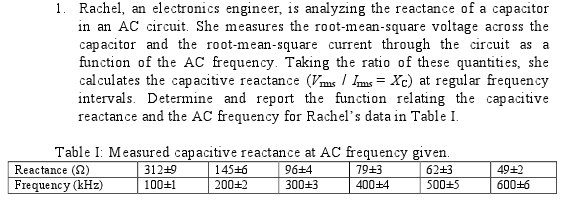## Homework Equations

For linear functions: y = Bx + A
For power functions: log y = n log x + log A
For exponential functions: ln y = Bx + ln A

## The Attempt at a Solution

I did actually graph the data first, with reactance as my independent variable (in the x-axis) and frequency as my dependent variable, and I'm guessing it's not an exponential function, just based on appearance.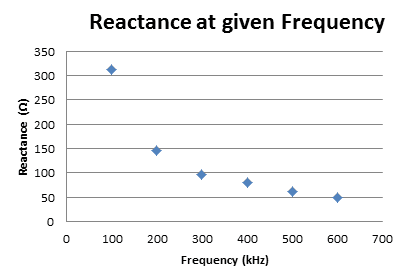I then tried plugging in my y values into power function equation, but I don't know how to derive the exponent n, or the coefficient A from that equation (assuming a power function relationship even exists in this case).

I'm hoping to get some direction here, so I can start on the other two data sets that I've also graphed.

## Answers and Replies

Mentor
Hint -- what does it look like if you make the horizontal axis logarithmic?

Youngster
Okay, just tried that and got a (nearly) straight line with a slope around -1.

So -1 would be n, and A (after some further calculation) would be about 4.492.

Plugged those in to check so that at x = 100, y = 310.7, and at x = 200, y = 155.34. These are pretty close, and sound reasonable when factoring in uncertainty. Thanks a lot!

Okay, I also managed to do the second one, but I need some clarification (and maybe help) with the last data table.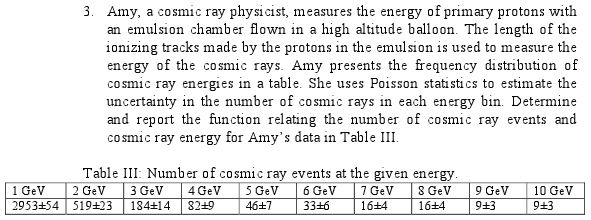The energy given by GeV is the independent variable here, yes?

Mentor
The energy given by GeV is the independent variable here, yes?

Yes. What does the graph look like?

Youngster
Alright, the graph looks like this: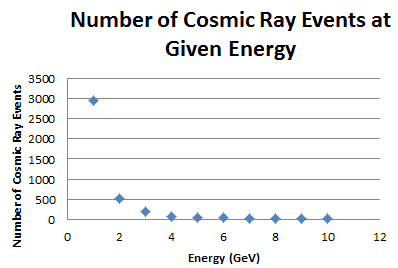And I was thinking this was an exponential function, but when I took the logarithm of both sides, I got another (somewhat) straight line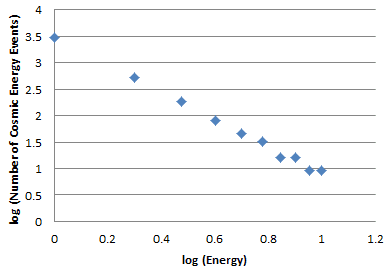So I'm guessing it's another power function since the natural log of the dependent variable does not form a straight line.

Plugging the values in, I again receive values pretty close to what should be expected. I suppose none of the data tables given provide an exponential function (the second one was linear when graphed).

Thanks a lot, I appreciate the help.

Mentor
Good work!